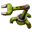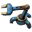## Understanding module calculations

Don't know how to use a machine? Looking for efficient setups? Stuck in a mission?
zOldBulldog
Smart InserterPosts: 1103
Joined: Sat Mar 17, 2018 1:20 pm
Contact:

### Understanding module calculations

I found a lot of information and even tools that calculate for you, but the information is a bit ambiguous and I could not find a single a clear example *in writing* of how to do the calculations.

For example, I tried to calculate the following.

Tier 3 PROD module = +10% items produced for a given product, -15% items/second
Tier 3 SPEED = +50% speed.

Refinery Basic Oil Processing with no beacons:
5 sec
100 Crude in = 20 Crude/sec
30 Heavy out = 6 Heavy/sec
30 Light out = 6 Light/sec
40 Petroleum Gas out= 8 PG/sec

So, 3 PROD modules in the refinery should result in:
1) 30% more items for the same inputs
Crude: 20 u/sec (same inputs)
Heavy: 6*1.3 = 7.8 u/sec
Light : 6*1.3 = 7.8 u/sec
PG: 8*1.3 = 10.4 u/sec
2) 45% fewer u/sec.
Crude: 20*0.55 = 11 u/sec
Heavy: 7.8*0.55= 5.29 u/sec
Light: 7.8*0.55= 5.29 u/sec
PG: 10.4*0.55= 5.72 u/sec

Then if I surround the refinery with 5 beacons in range on each side and each holds 2 speed modules (20 speed modules = 10*2*50% = 1000%):
Crude: 110 u/sec
Heavy: 52.9 u/sec
Light: 52.9 u/sec
PG: 57.2 u/sec

I suspect that the above calculation is somehow wrong.

The reason I think this is that most designs use 10 refineries and 57 pipes from input to input, so assuming a pump right before right before the 1st refinery, that should result in a pipe throughput between 1067 and 1033 according to the Maximum Flow table (https://wiki.factorio.com/Fluid_system), and that is less than 110*10=1100 u/sec required in inputs.

So,

1) Where is the error in my calculations?

2) Do you know of a link to a page where I can see the proper way to calculate u/sec correctly and presented in an easy to understand format/example?

3) If you can't answer either of the above, could someone show me how to calculate u/sec in the example above using the same modules I used?

Thanks.

Xeorm
Fast InserterPosts: 150
Joined: Wed May 28, 2014 7:11 pm
Contact:

### Re: Understanding module calculations

Main thing to remember with modules is that the speed changes are additive.

Take a refinery. It has a crafting speed of 1. Crafting speed of crude oil is 5 seconds. Default speed is 5 / 1 = 5.

Add some modules. By themselves, productivity modules have -15% speed. 3 of them add up to -45%. Our new recipe time is 5 / .55 = 9.09.
Then multiply by 1.3 to find the output. Same as your initial values.

Add the beacons next. Each beacon has 2 speed modules, but their effect is half that of normal due to being in a beacon. 10 beacons means you have 10 * 2 * 50% / 2 = 500% speed boost. This is additive with the earlier speed boost. 55% + 500% = 555%. 5 / 5.55 = .9.

Then run earlier numbers.

crude: 100 / .9 = 111.1
heavy: 30 / .9 * 1.3 = 43.3
etc.
Rearrange numbers as you need to for what feels comfortable.

### Who is online

Users browsing this forum: Google [Bot]